## 4.17External wall heat ﬂux

The boundary condition for temperature (or energy) dictates the heat transfer across a boundary. At a boundary that represents a solid wall, simple conditions can sometimes be applied. However, specialised boundary conditions are often required that control the heat ﬂux across the boundary.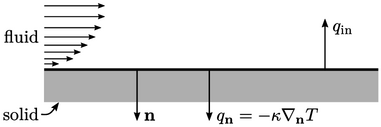The ﬁxed temperature is the simplest condition, setting a ﬁxed value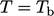. This condition provides an approximation for cases for a solid with high thermal mass, due to a large mass of material and high conductivity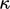, which helps to maintain constant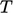.

Otherwise the boundary condition sets the heat ﬂux normal to the boundary,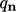derived from Eq. (2.54 ) by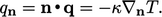(4.28)
Another simple condition is zero gradient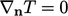. This is the adiabatic condition, corresponding to zero normal heat ﬂux by Eq. (4.28 ), suitable when the solid is a thermally insulating material with a large mass and low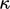.

Otherwise a ﬁxed heat ﬂux condition speciﬁes an inward heat ﬂux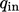as a ﬁxed gradient type with a reference gradient by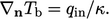(4.29)

### Fixed heat transfer coeﬃcient

Another way to specify the heat transfer at an external wall is by Newton’s law of cooling.7 This general law states the rate of heat loss of a body is directly proportional to the diﬀerence between the body temperature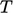and a surrounding, ambient temperature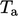.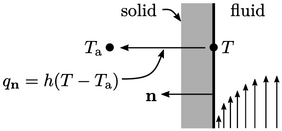Applied as a boundary condition,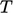is the ﬂuid temperature at the boundary, and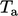a temperature some distance beyond the solid boundary. A heat transfer coeﬃcient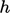, with SI units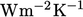, provides the constant of proportionality such that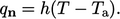(4.30)
Substituting Eq. (4.28 ) and rearranging gives an equation for the ﬁxed heat transfer coeﬃcient condition: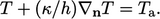(4.31)
The equation has the form of a Robin condition, Eq. (4.10 ), so can be implemented as described in Sec. 4.9 . The coeﬃcientis typically characterised for the particular ﬂow regime and solid boundary, by some estimate, experimental measurements or computer simulation.
7Isaac Newton, Scala graduum caloris. Calorum descriptiones & signa, Philosophical Transactions, 22:270, 1701.

Notes on CFD: General Principles - 4.17 External wall heat ﬂux